# THE EARTH'S CORE IS OVERHEATED EMPTINESS

The Earth will be blown up by the scum, who call themselves as scientists. Society you are a stupid cattle - because, despite the existence of this and similar web-sites, you did nothing for the detention of terrorists, physicists from CERN and other scoundrels, sitting in academies and promoting this blasphemous experiment.

September 11,  2010.

uuu uud udd ddd
uus uds dds
uss dss

sss

These are triads, which can be made of up, down and strange quarks. One of these triads is stable in isolated state; it is a proton, uud. All other triads are more massive than proton (uud), as a result, they all decay. It is known, that the triad udd, which is neutron, decays, if it is isolated. The cause of decay is clear; - the mass of free neutron is bigger than the mass of free proton.

But the situation is different inside another environment:
1. inside the neutron-excessive nuclei a neutron decays at proton, electron, electron antineutrino;
2. inside the stable nuclei the number of neutrons and protons do not change;
3. inside the neutron-deficit nuclei a proton decays at neutron, positron, electron neutrino.

This shows us, that sometime a triad udd can be more stable than uud triad. Now we can make a conclusion: stability of a quark triad depends on the properties of a subnuclear condensate.
The question arises, - is there a subnuclear condensate, where some quark triads be more stable as usual proton-neutron condensate, which are the nuclei of our Earth?
Till now physicists had received unstable nuclei, consisting from three types of triads: protons (uud), neutrons (udd), and lambda-hyperons (uds).

Here are some examples of such hyper-isotopes:
hyper-hydrogen (pnl), (pnnl);
hyper-helium (ppnl), (ppnnl), (ppnnnl), (ppnnnnl), (ppnnnnnl), (ppnnll);
hyper-lithium (pppnnnl), (pppnnnnl),(pppnnnnnl), (pppnnnnnnl).

Till now physicists had received even an anti-hyper-hydrogen, which is also unstable.

But look at the beginning of this text, and try to understand, when unstable free neutron becomes more stable than proton, if neutron is not already free, but bonded in subnuclear condensate.

Try to answer, what will happen with the ordinary matter, if physicists will create a stable condensate of lambda-hyperons, or of a mixture of neutrons and lambda-hyperons.

These subnuclear condensates have no limit of grows. Usual subnuclear condensate, consisting of protons and neutrons, do, have the limit, which is uranium. Uranium finishes the periodic table of stable elements. The existence or absence of the limit is explained by the electrical charge of a condensate. Usual nuclei have an electrical charge. Condensates, made of lambda-hyperons and/or neutrons, do not have the electrical charge. As a result, they do not have Columbian barrier, which do not let the ordinary nuclei to fuse.

The 9-th of November 2010 CERN plans to collide heavy ions. As a result, the Solar system can be exploded into a nova star. If strangelets will capture ordinary nucleons, ruin them and grow without decay on smaller strangelets, then the duration of explosion will be about of 500-1000 days. If growing strangelets at some moment would decay at smaller strangelets, then the duration of Earth explosion can be much less.

There are some other microscopic condensates, which can be created at LHC, for example, microscopic magnetic holes. Result of such experimental discovery will be the same – the Earth’s explosion.

Heavy ion collision is a new dangerous step, planned by CERN physicists. They will be started in two months. Politicians, businessmen, ordinary people believe that “the best physicist” on the planet are correct. CERN propaganda had made its criminal role; - courts, switched on by opponents of dangerous CERN’s experiments, are failed.

So, people, be ready to be murdered. You did not believe us earlier, you do not believe us now. You a lazy to ruin the CERN, you are lazy to strike in order to save yourself. You are a crowd of silent cattle, which must be killed. I wish you happy flight in icy comets to other star systems. Go and bring there a seeds of new life. Do not fear to die, and fulfill your sacred mission. Fasten your safety belts; - be ready to suicidal reproduction of biospheres.

September 7,  2010. From my point of view, the most dangerous things, which can be made on proton-proton (p-p) collisions at powerful colliders, are two different condensates: microscopic magnetic holes and microscopic droplets of strange matter. In the bottom I’ll name both of them by unifying term “droplets’.

I have made a program "Explosion of the Earth, switched by criminal physicists" and want to show you some results.

The time interval of the Earth explosion depends from several parameters: the number of created droplets; the density of matter inside droplets; the velocity of particles in plasma bubbles around the droplets.

Let density of droplet will be 300 times greater than nuclear density;
Let velocity of particles in the plasma bubbles will be v=c/10.

Then:

a. if there were created 100 000 000 000 droplets, then the Earth will be exploded in 6 days;
b. if there were created 1000 000 droplets, then the Earth will be exploded in 272 days;
c. if there were created 1 droplet, then the Earth will be exploded in 75 years.

Result "c" can be rejected. Physicist will not stop if they will see one unusual track.
Result "a" can also be rejected, because it is impossible to create 100 000 000 000 droplets in the very first days.

In the upper results we supposed that the droplets were made simultaneously.
Now we'll remade the program and let’s suppose that they are created continuously:

a. 10 droplets per second;
b. 1 droplet per second;
c. 1 droplet per 10 seconds;
d. 1 droplet per 100 seconds.

Then the Earth will be torn on fragments in

a. 98 days;
b. 175 days;
c. 311 days;
d. 552 days.

As we know LHC works with quite long stops. So, I think, that the option "d" is the most reliable. As a result, I supposed that the total time of Earth explosion into fragments is about 1000 days.

Several more interesting results:
1. The mass of Earth’s matter, ruined before the rest of it would start into cosmos with velocity about 10 km/s, is about 1015 kg, - that is about one billionth part of Earth. That also will be approximately the total mass of all droplets.
2. The Sun will be ruined in about a 1000 years. The Sun will undergo several successive explosions as nova. By the way, that explains the rings of SN 1987A.
3. The energy output of matter’s collapse at droplets at the time of “Earth torn on peaces” will be about the luminosity of the Sun. That means that neutrino flux from the Earth at that time will be by (R/r)2 times bigger than today’s neutrino flux from the Sun. (R – radius of Earths orbit; r – radius of the Earth). The graph of heat power in the “continuously” case is a cubic parabola; consequently, we are able to register the excessive flux of neutrino from the Earth already now! If there is no such excessive flux, then I was wrong. But do not forget about future ion-ion collisions; do not forget about future 2*7 TeV p-p collisions. Those are other possibilities to kill the Earth, to kill the humankind.

CERN and all corrupted science are responsible for the probable future murder of 6 billion people and probable destruction of the Earth.

It was subscribed in the Universal Genetic Code of Eternal Living Universe that we, the enemies of biosphere's suicidal reproduction must be undergone to ban, to laughter and shame; otherwise the reproducing act would not occur. That is the law of biosphere's reproduction. So, don’t fear to die and perform your sacred mission. Fasten your safety belts; - be ready to biosphere’s suicidal reproduction!

Appendix: If you do not trust to my computed results, you can compose your own program and test it. My program works by small steps, measured by time interval, for example, dt=1000 seconds. At every step it computes the number of ruined protons by this formula dN = dt v 4pr(i)2k / 6, where v = c/10, c – velocity of light; 4pr(i)2 – the surface of a droplet at i-th step; r(i) – radius of a droplet at i–th step; k – concentration of protons in a plasma bubble around a droplet, k = MEarth/((4/3)pREarth3) /  mproton. The number “6” in a formula for dN is explained by the fact that our space has 3 dimensions and by the fact that only 1/2 of particles move in positive direction.
Radius of a droplet at the next step: r(i) = r(i - 1)((N + dN) / N)1/3.
The total number of ruined protons at every step: N = N + dN.
Heat power at every step, if droplets are made continuously:
P(i) = P(i - 1) + dN mproton c2 / dt / 2 * Ndr;
P(i) = dN * mprotonc2 / dt / 2 * Ndr.
In “simultaneously” case P-graph is square parabola; in “continuously” case P-graph is cubic parabola;
Ndr – number of droplets created per step.

The program stops, when the total heat output, Q, becomes bigger than 3*1032 Wt. This amount of energy is enough to send all matter of the Earth to infinity. Inevitable energy losses can be neglected if T < 1000 days. If T > 1000, then the true value of T will be the more, the more it’s computed value.

Note 1. It was supposed that every proton, p, colliding with microscopic magnetic hole, NS, decays and the droplet becomes bigger. NSn + p = NSn+1 + e+, where: e+ - positron; subscripts n and n+1 are the number of x-bosons in the magnetic hole; x-boson is a smallest magnetic excitation in ferromagnetic Savidy vacuum, ns. As a result of proton capture the region around magnetic hole will have an excess of neutrons. That will lead to beta decays and to flux of electronic antineutrino from that region.
Note 2. It was supposed that every proton, p(uud), and every neutron, n(udd), colliding with droplet of strange matter, N(uds), decays and droplet becomes bigger. N(uds) + p(uud) = (N+1)(uds) + K+, where: K+ - positively charged kaon. (N) and (N+1) are the number of lambla-hyperons in a growing strangelet. Lambla-hyperon is a strange nucleon (uds) in a strangelet N(uds). A strangelet can capture neutrons too: N(uds) + n(udd) = (N+1)(uds) + K0, where: K0 - neutral kaon.
K+ and K0 decay. As a result an additional flux of different sorts of neutrino and antineutrino can be registered.
Every capture of nucleon by a droplet is accompanied by heat output of about half of rest energy of nucleon. That means that the binding energy of a droplet is extremely high; that specific energy output of nucleon collapse onto droplet is hundred times more than the specific energy output in uranium or hydrogen bombs. But the time interval of collaptical explosion is quite big, because, in order to explode the Earth into peaces, the droplets must successively grow from 10-23 kg to 1015 kg / Ndr.

September 3,  2010.

To read more on this topic open the page Collider, News, Links.

Read articles Supernova ejects material asymmetricallyand CERN faces €250m budget cuts and my recent commentaries to those articles.

April 2,  2010. Look at the patterns at the page Bureau of Meteorology images show mysterious patterns on radar system.These patterns are beautiful. And magnetic wasps are better than the whole Earth explosion...

To read more on this topic open the page Collider, News, Links.

March 30,  2010. Crime had happened. Collisions with the energy 3.5 TeV per proton or 7 TeV per collision were performed.

To read more on this topic open the page Collider, News, Links.

March 19,  2010. Today night the criminals from CERN accelerated protons to the record energy 3.5 TeV per beam. At the regions of collisions, probably, the rays were on skew lines (two lines that do not intersect but are not parallel). It is not excluded that there were accidental collisions of protons.

If the collapse was switched, then most probably tomorrow morning all people will start to cosmos.

According to plan the first collisions at 3.5 TeV must be fulfilled the 30-th of March.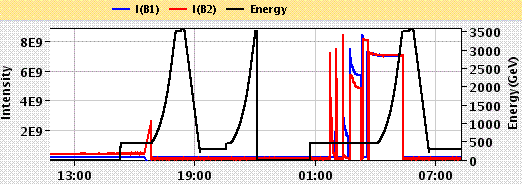March 10,  2010. By the way, it seems, unstable condensates were already been created at Tevetron. That follows from the multimuon events (up to 8 per droplet and antidroplet) described at the end of 2008 by CDF collaboration.
From the other hand, some authors say that "strange condensate" is stable if it has more than 10 lambdas, 10(uds).

Here is a figure from one Russian-language page about those events.c) – Abnormal events. I would change the signs “?” by “N(usd)” and “N(usd)”.

The next figure is taken from the article RHIC nets strange antimatter. (Read my commentary there.)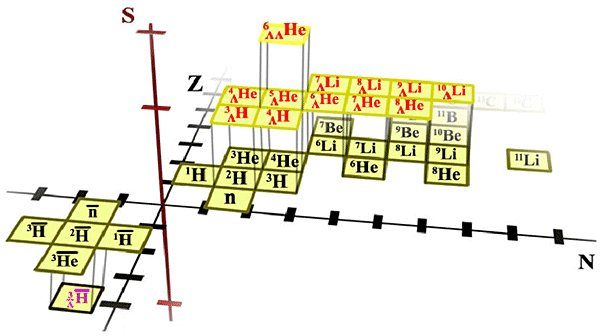...

To read more on this topic open the page Collider, News, Links.

...

...This portrait is dedicated to CERN decision to start collider work the 20-th of February with energies 3.5 TeV per proton....

...

Bell paradox. If two rockets, connected by a string,..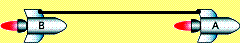...

...These formulae give Unruh/Hawking temperatures at the depth R, which is equal equal to Compton radius of a particle Rc...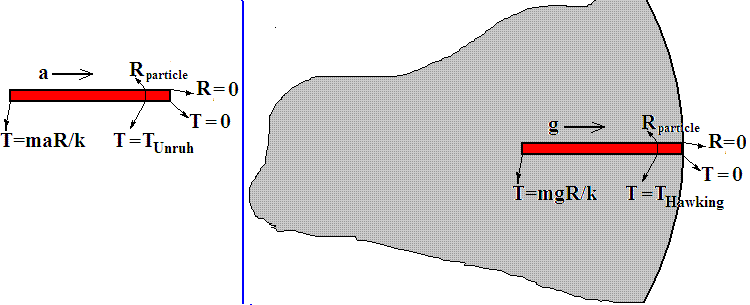...

... This is not my picture. Perhaps its author is my good friend, supporter.Citizens! Do not think that some good Uncle Ivan would come and destroy the CERN and its doomsday machine, LHC. Think yourself for your salvation. And not just think, but act. I have no flight experience, no money for the fighter and for a nuclear warhead to bomb the LHC. If you will not stop the CERN now, the CERN will kill all of you in the first quarter of this year, with the probability of about 50%.

...

Proton-proton collision, which leads to creation of stable excitation of physical vacuum, can look like this inelastic collision.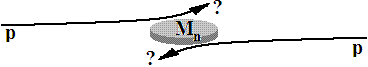Directions of both protons were changed...

...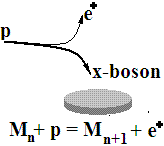... In the ordinary vacuum different virtual particles constantly appear and disappear. In the bottom figure we can see the appearance of electro-positron pair in the point A and their annihilation in the point B.

...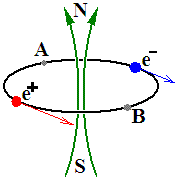In order to transform virtual pair into real pair we must spent energy. On the next figure we can see the x-boson, consisting from two fermions, particle and antiparticle.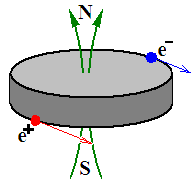If the energy of p-p collision at collider is sufficient we can create an exited region in vacuum containing some quantity of such x-bosons. They are oriented in the same direction and have the superconductive and ferromagnetic properties.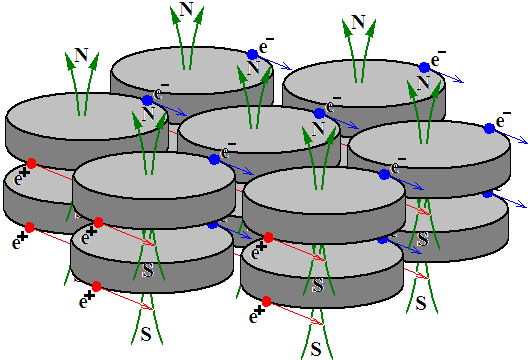If proton enters into the field of such exited vacuum it can be ruined onto x-boson and positron...

...

To read more on this topic open the page Collider, News, Links.

Reproduction of biospheres and civilizations.

Arguments,

Crude errors in the LHC safety documents. What will LHC give us, the particle of God, or magnetic trap of Devil?

The end of extremely urgent note.

# THE EARTH'S CORE IS OVERHEATED EMPTINESS

Any massive object: the Sun, the Earth, a star, a planet, a nuclei of galaxies and quasars are empty inside. This emptiness is filled with rarefied gas and radiation at high temperatures. There are two causes leading to formation of the emptiness. The first cause is the pressure of photon gas. The second cause is the Arc Effect discovered in 2000. On the bottom figures you can see the dependence of some physical values from the distance to the center of the objects. We'll investigate the objects of different masses. The graphs were received with the help of a computer program, which integrates the system of differential equations. I made it in the year 2003 and developed this year (2007). On the right side I'll place the graphs, received according to classical equations, on the left side I'll place the graphs received according to my equations, accounting the Arc Effect.

#### The Sun-like object, M = 1MSun; R = 1RSun; mparticle = 1.5 a.u.m.

 Our model Standard model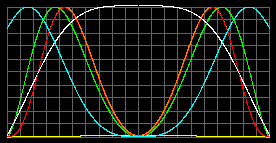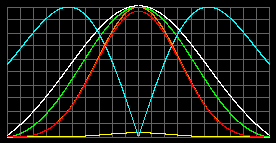Tcenter = 7.66 millions K. Total pressure pmax =8.4*108atm at r=0.56R Density rmax=2.5 t/m3 at r=0.632R Tmax=18 millions K. pmax =7.96*109atm at center. rmax=7.8t/m3

Green - concentration of particles; red - gas pressure; yellow - radiation pressure; orange - total pressure; white - temperature; blue - acceleration g. Graphs were normalized to the scale of window and surface gravity acceleration in both cases are equal.

#### Object with M = 10MSun; R = 50RSun; mparticle = 1.5 a.u.m.

 Our model Standard model ruined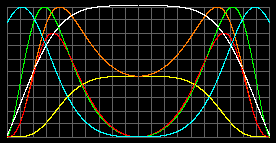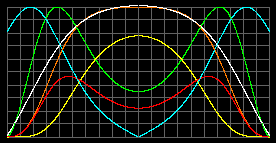Tcenter = 1.2 millions K. pmax =1.2*104atm at r=0,6R. rmax=0,19 kg/m3 at r=0,717R Tmax=1.77 millions K. pmax =31519atm at center. rmax=0,2kg/m3 at r=0,617R

As we can see the radiation had naked the center in both cases. The pressure and particles concentration is not sufficient for nuclear fusion in such stars. Mainstream astrophysicists slander us. Or try to hide the radiation pressure under their carpets.

#### Object with M = 70MSun; R = 500RSun; mparticle = 1.5 a.u.m.

 Our model Standard model become as ours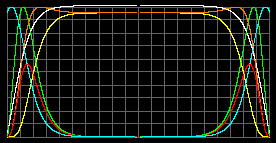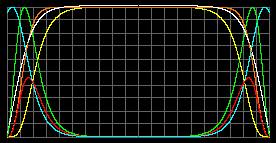Tcenter = 363 thousands K. pmax =45atm at r=0.74R. rmax=1.86 g/m^3 at r=0.88R. Tcenter = 391 thousands K. pmax = 58atm at center. rmax=1.81 g/m3 at r=0,87R.

Pay attention at the values. Nuclear reactions are impossible for giant stars.

Let's diminish the radius, for example at ten times (object with M = 70MSun; R = 50RSun; mparticle=1.5 a.u.m.). We'll have almost the same graphs but the temperature will rise ten times, common pressure will rise in 104 times, because it now dependent mostly of radiation. And the density will rise in 103 times. That is also insufficient. Energy sources of standard astronomy disappeared.

But we have no need to take that to heart. We know the other sources of star's energy. And we need to know the real non-falsified internal structure of stars. Let’s now look at the objects, smaller then our Sun.

White dwarf, Object with M = 1MSun; R = 0.01RSun; mparticle = 2a.u.m. Density of condensation of gas into liquid (degenerated gas) = 2*109 kg/m3.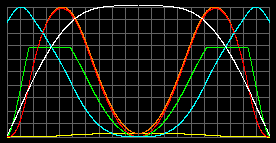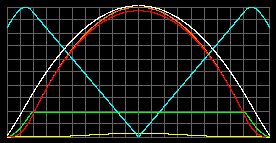Tcenter = 920 millions K. pmax = 6.9*1016atm r=0,581R. The liquid state with rmax=2*109 kg/m3 stretches from 0.83R to 0.52R. Tcenter = 1249 millions K. pmax =2.1*1017atm at center. The liquid state with rmax=2*109 kg/m3 stretches from r=0,81R to center.

Pay attention on the temperatures! But this is not so extraordinary if we mention that surface temperatures of some white dwarfs are 10000 K, 60000 K, and higher. (The difference in heights of green graphs is the result of program normalization. In fact we have launched the same density of condensation of gas into liquid.)

#### Jupiter-like object: M=0.00095MSun; R = 0.1025RSun; mparticle = 4a.u.m. - was taken arbitrarily.

Let's take arbitrarily the density of condensation of gas into liquid equal to 2000 kg/m3 for our equations, and 6000 kg/m3 for standard model.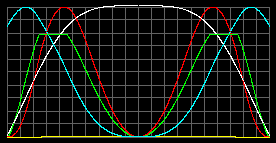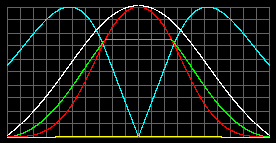Tcenter = 183 thousands K. pmax = 6.4*106atm at r=0.57R. The liquid state with rmax=2000 kg/m3 stretches from 0.76R to 0.54R. Tcenter = 444 thousands K. pmax = 6.4*107atm at center. The liquid state with rmax=6000 kg/m3 stretches from 0.25R to center.

#### The Earth-like object: M = MEarth; R = REarth; mparticle = 20a.u.m. - was taken arbitrarily.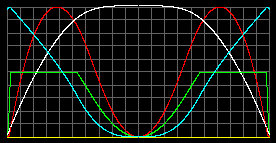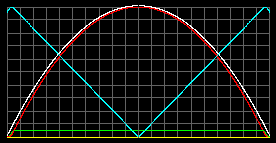Tcenter = 25 thousands K. pmax = 6.6*105atm at r=0.62R. The liquid/solid state with rmax=6000 kg/m3 stretches from 0.97R to 0.47R. Tcenter = 33 thousands K. pmax = 1.9*106atm at center. The liquid/solid state with rmax=6000 kg/m3 stretches from 0.95R to center.

The difference in heights of green graphs is the result of program normalization. In fact we have launched the same density of condensation of gas into liquid/solid, r=6000 kg/m3.

The received values of temperature surpass the values, proposed in literature, somewhere about 5 times. The central temperature can be decreased by decreasing of the coefficient of temperature gradient. In all upper cases we have used the coefficient 2/5, which is reasonable for gaseous medium, but is controversial for liquid/solid medium. Let's diminish it to 1/10 and try again.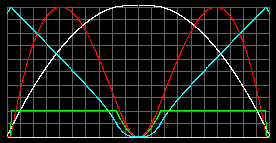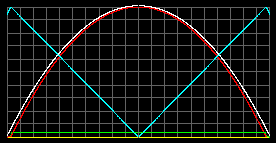Tcenter = 7.9 thousands K. pmax = 7*105atm at r=0.59R. The liquid/solid state with rmax=6000 kg/m3 stretches from 0.97R to 0.17R. Tcenter = 8.2 thousands K. pmax = 1.9*106atm at center. The liquid/solid state with rmax=6000 kg/m3 stretches from 0.97R to center.

Let's analyze the graphs in our model. The drop of pressure begins approximately at r=0.6R, but the temperature is already quite high there. That means that there must exist the point of transition of substance from solid state into liquid. It is known from seismology that the border between the core and mantle is situated at r=0.54R. That border strongly reflects P and S waves and refracts the P-waves. Our graphs of pressure and temperature allow us to divide the horizontal graph of density on two regions: solid and liquid. But standard model graphs do not obey us to do that, - the continuing growth of pressure rises the temperature of melting. At last, lets analyze the point of transition from liquid state into gas. It is the boundary between the external and internal core. Standard geology says that external core is liquid and internal core is solid. We say: the external core is liquid; the internal core is gaseous. We can see there the crack at the density graph. But it is known from the thermodynamics that at high temperature there is no distinct boundary between liquid and gas. There must be a smooth transition. Consequently, I'll paint by hand the region of liquid phase in dark-blue color. I'll smooth the crack and paint the gas phase into yellow color.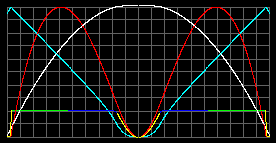The external core (dark-blue) is liquid and keeps the S-waves out; the internal core (yellow) is gaseous and keeps both waves out.

### Used equations.

If we do not account the light's pressure and mass the following systems is valid.

 With Arc Effect. Standard-like equations. dp/dr = - gr + 2p/r. dT/dr = - (2/5)mg/k. dM/dr = 4pr2r. g = GM/r2. p=nkT. r=nm. dp/dr = - gr. dT/dr = - (2/5)mg/k. dM/dr = 4pr2r. g = GM/r2. p=nkT. r=nm.

If we account the light's pressure and mass the following systems is valid.

 With Arc Effect. Standard-like equations. d(p+p')/dr = - g(r+r')+2p/r. dT/dr = - (2/5)mg/k. dM/dr = 4pr2(r+r'). g = GM/r2. p=nkT. r=nm. r'=u/c2. u=3p'. u= 4sT4/c. d(p+p')/dr = - g(r+r'). dT/dr = - (2/5)mg/k. dM/dr = 4pr2(r+r'). g = GM/r2. p=nkT. r=nm. r'=u/c2. u=3p'. u= 4sT4/c.

If we take into account the density of condensation of gas into liquid/solid, the p=nkT or n=p/(kT) is changed into:

 n = p/(kT) if p/(kT) smaller than nmax. n = nmax. if p/(kT) biger than nmax.

In the case of Earth we tried two variants for temperature gradient:
dT/dr = - (2/5)mg/k and dT/dr = - (1/10)mg/k.

I saw two types of equation of hydrostatic equilibrium.

dp/dr = - gr, for ideal gas object.
d(p+p')/dr = - g(r+r'), for ideal gas object filled with radiation.

From my point of view these equations lead to errors because they do not account the Arc Effect. These equations are correct for the object filled with non-colliding particles, for example, for star congestions filled be stars, for photon gas enclosed in some vessel, for very rarefied gaseous object with particles having the length of free pass, greater than the object's radius.

If we account the collisions between particles, we'll have the following equations:

dp/dr = - gr + 2p/r, for ideal gas object.
dp/dr = - gr + 2(p-p0)/r, for ideal gas object, enclosed by the shell with pressure p0.
d(p+p')/dr = - g(r+r') + 2p/r, for ideal gas object filled with radiation.
d(p+p')/dr = - g(r+r') + 2(p-p0)/r, for ideal gas object filled with radiation, enclosed by the shell with pressure p0.

Pay attention that the last item in these equations does not contain the radiative part of pressure, p'. The cause is clear, - the light does not obey the Arc Effect, because the light beam do not change the direction of another light beam at their cross-point; the photons can exert pressure upon matter screen, but photons do not exert pressure upon other photons.

The computer modeling had showed me, that the usage of the symmetrical equation, containing p' in the last item, leads to appearing of a hole in the center of the object. The more the temperature of the object is the more the hole.

You can try my computer program Arc Effect - 2007. It is uploaded at "narod/ru" and at "webcenter", exe-files, 70kb.

March, 2007.

#### Text from May 2000.

Notes from March 2007 I'll paint in yellow.

Any massive object: the Sun, the Earth, a star, a planet, a nuclei of galaxies and quasars are empty inside. This emptiness is filled with rarefied gas and radiation at high temperatures. In average, at constant radius, the more massive object is, the more temperature is inside of its depths...(cut). On the other hand, the further a star on a scale of evolution, is the thinner its shell. The rupture of a star shell leads to the explosion of nova or supernova. (...) To understand these paradoxical conclusions let's try to analyze the dependence of a pressure from the distance to the center of an object.

Let’s assume at first, that the massive object is a giant gas ball. (...) Let’s break a star into a series of concentric layers. The gravity force pulls each layer to the center of the object; and its weight dP is transferred to a bottom layer. Having divided the weight by the area of sphere between these spherical layers we shall receive an increment of a pressure, which is added to the whole pressure, transmitted from the top layer to bottom: dp = dP / S. In order that the object be in balance it is necessary, that the pressure of gas and radiation in the bottom layer be greater than the pressure of gas and radiation in the top layer by the quantity: dp = pi+1 - pi. The expression dp = dP / S is the equation of hydrostatic equilibrium. It can be rewritten in the form:

dp = dm * g / (4pr2) = -r * g * dr,
or shortly: dp/dr = -rg. (1)

where: r is a density on a distance r from the center of an object; g is an acceleration of free fall for given r; dm is a mass of a layer.

dm = - r * S * dr, (2)

(When we move from outside to the center, dr is negative, consequently, dm and dp are positive.)

Alas, the equation (1) is only fair for a homogeneous gravitational field, and for the object with non-colliding particles. It is not applicable to a spherically symmetric gravitating object. This mistake leads to a grandiose growth of pressure and density inside of planets and stars. So as the equation of hydrostatic equilibrium for spherically symmetric objects does not exist, or I did not met it earlier, it is necessary to deduce it here, proceeding from elementary principles. (That was my first proof of Ark Effect, logical proof. Several years later I have found another proves: analytical, mathematical, historical, computer modeling). So, on page the description with the help of figures 2-6 it was shown, that the formula (1) should not include the acceleration of a free fall g, but it must include the value v02/r - vt2/r. Thermal velocity vt coincides with the most probable velocity vpr. If we use the average squared velocity v, than: v02/r - vt2/r = v02/r - (2/3)v2/r. Let's name this value the absolute (or internal) acceleration of free fall, ga.

ga = v02/r - vt2/r = g0 - gt, (3)

where: v0 – orbital velocity of a particle for a given r; vt – average thermal velocity of a particle; g0 - Newtonian acceleration of free fall, or external acceleration of free fall, acceleration of free fall in vacuum. For Newtonian acceleration of free fall we can write:

g0 = g0M/r2, (4)

where: g0 - gravitational constant, renamed here as external gravitational constant, or gravitational constant in vacuum. The similar expression can be written down for absolute (internal) acceleration of free fall, acceleration of free fall in medium, without friction:

ga = gaM/r2 = g0 - gt = g0M/r2 - gt, (5)

Having divided (5) on M/r2 we shell receive:

ga = (g0 - gt )/(M/r2) = g0 - gtr2/M, (6)

Let's take the ratio of ga to g0:

g = ga / g0 = (g0 - gtr2/M) / g0 = 1 - gtr2/(Mg0) = 1 - gt/g0 = 1 - (vt/v0)2, (7)

Outside of the object, or far from the object, or in the vacuum cavity inside the object, the probe body will be drawn to the object with the force:

F = g0Mm/r2, (8)

The element of the object itself with the same regional density, which has the same temperature as the object, will be drawn to a part of the object, situated under a probe body, with the force:

F = gaMm/r2 = gg0Mm/r2, (9)

Let's compare expressions (8) and (9) to the Coulomb law for vacuum (10) and for medium (11):

F = (1/(4pe0)) * Qq/r2, (10)

F = (1/(4pee0)) * Qq/r2 = (1/(4pea)) * Qq/r2. (11)

As we can see, the role of permeability of vacuum, or the role of the electrical constant e0 plays the factor g0; the role of relative permeability e plays the factor g, the role absolute permeability of medium ea plays ga.

To these strange analogies it is possible to add the following ones from electrodynamics:

em = (c/v)2; or if m = 1, then: e = (c/v)2, (12)

And its analogue, the equation (7):

g = ga / g0 = 1 - (vt/v0)2.

Consequently, ga is a gravitational constant, applicable to a body, situated in a spherical layer of object, where the molecules of the layer have the average thermal velocity vt, and with the same regional density. The average squared velocity can be defined from the equation: mv2/2 = 3/2*kT where: m - weight of a molecule of air; T - the temperature of gas (...). The value vt can be find from equation vt2 = (2/3)v2.

And g0 is the gravitational constant in vacuum, which is applicable to a probe body far outside of attracting object.

At last, g is a relative gravitational permeability of a medium.

The equation g = ga / g0 = 1 - (vt/v0)2 for the object with density, which is not equal to the regional density of the object, will have the coefficient robject/rprobe. But main profit of this approach is in the demonstration of symmetries between the Newton and Coulomb laws. In fact such record of the formulae unites the attraction force in vacuum and repulsion force of Archimedes.

#### Stop correcting.

I don't correct the further 2000-text. I did not solve the riddle of my excellent formula G' = 1/exp(a + 1/a) yet.

Let's now return to a fine structure constant a, which is the running constant of the electromagnetic interactions, and to the early received gravitational constant, which in the normalized units is dimensionless, denoted there by G'; and it is the gravity fine structure constant:

G' = 1/exp(a + 1/a), (13)

The transition to SI units can be carried out under the formula:

G = (G'e3/(4sqr(a)e03/2melmpr2))2/3. (13 a)

From this formula G is equal to 6.671479888E-11 N*m2/kg2, that is in the perfect consent with the experimental value of G. According to CODATA-1999 the experimental value of G lays between 6.663E-11 and 6.683E-11 in SI units. According to CODATA before 1999 the experimental value of G was: (6.67259E-11 + /- 0.00085E-11) N*m2/kg2. There is a question, why the accuracy of G has fallen? There is the second question, why for normalized units the formula 1/G ' = exp(a + 1/a) works, instead of, for example, the easier one: 1/G ' = exp(1/a)?

The answer consists in the following:
The quantity a can be regarded as the velocity of an electron in an atom of hydrogen, measured in the shares of the velocity of light.
The quantity (1 - a2) – is the relativistic amendment, which can be represented as (1-(v/c)2).
The ratio of quantities exp(1/a) and exp(a + 1/a) with good accuracy is equal to (1- a2) or to (1-(v/c)2).

Electron can be represented as a cloud, as an atmosphere of an atom, for which the gravigeometrical paradox works too.

That is why, the quantity G in old denotation, or g? in new denotation is also the running constant. The expressions exp(1/a) and exp(a+1/a) just also must give 1/ga' and 1/g0'. But what correspondences between them are? Preliminary and, while what not the final analysis, gives:

It is obvious, that g0 should be greater than ga.

Hence, g0' should be greater, than ga'.

Hence, 1/g0'should be less, than 1/ga'.

(1/a) is less, than (a+1/a), and they both are more than 1.

Consequently: exp(1/a) is less, than exp(a+1/a).

At last we conclude, that:

1/g0' = exp(1/a), or g0' = 1/exp(1/a), (14)

1/ga' = exp(a+1/a), or ga' = 1/exp(a+1/a), (15)

Let’s try these results in SI units:

g0= (g0'e3/(4sqr(a)e03/2melmpr2))2/3 = (e3/(4sqr(a)e03/2melmpr2))2/3*(exp(1/a))-2/3, (16)
ga = (ga'e3/(4sqr(a)e03/2melmpr2))2/3 = (e3/(4sqr(a)e03/2melmpr2))2/3*(exp(a+1/a))-2/3, (17)
g = g0/ga = (exp(a+1/a))-2/3/ (exp(1/a))-2/3 = 1/exp(2a/3) = 0.995146913, (18)

g0 = 6.704015058E-11 N*m2/kg2,
ga = 6.671479888E-11 N*m2/kg2.

In the expression (18) the relative gravitational permeability of a medium can be received through a constant a with good precision. On the other hand, we can check up this result applying the formula (7): g=1-(vt/v0)2. In order to do this we must know the value of the orbital velocity: v0=Sqr(g0M/r) and the value of average thermal velocity of molecules of the air near the surface of the Earth vt = Sqr(3kT/(29*a.u.m.)), where a.u.m.  – atomic unit of mass, 29 - molar weight of the air; and let T be equal to 300 K. Then:

v0=7910 m/s; vt = 508 m/s; G = 0.995876

This result does not absolutely coincide with the result of the formula (18) for several reasons:

1. The temperature 300 K is not very much approached to the real value of temperature. The check has shown, that for planets and stars we can receive rather good results.

2. The reason of discrepancy is contained also in that, that the sole value G, used in physics and astronomy now, actually in one cases "works" as ga, and in the others - as g0. So, between planets and stars the value g0 works, but in the ground laboratories at pumped out air the value g0 works, and at presence of the air the value ga works. Last two items are correct, if we measure the force between the Earth and the probe body. But actually, the mass of the Earth is unknown, and we measure G between two probe bodies, between which the additive item g0 works. All this confuses a situation at all.

Despite of these difficulties, it is necessary to move forward in the study of gravitation. This will unite the gravitation with electromagnetism. It, at the end, will simplify the understanding of gravitation. The experiment with the Gravity-Thermal Coil is extremely necessary. This experiment will show us the structure of the depths of planets and stars. This experiment will allow us to solve many energetic, ecological and technical problems.

The Arc Effect.

Program Arc Effect is uploaded at "narod/ru" , 70kb.

To the index of Space Genetics

My Curriculum Vitae.

Ivan Gorelik.